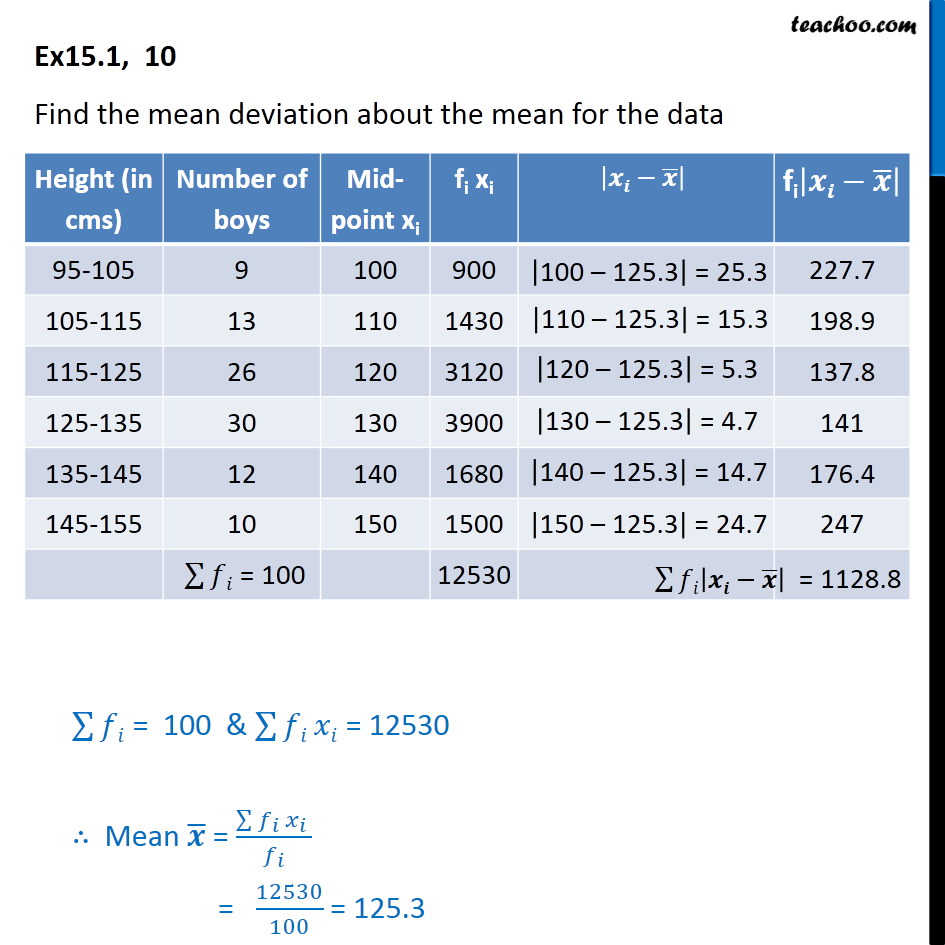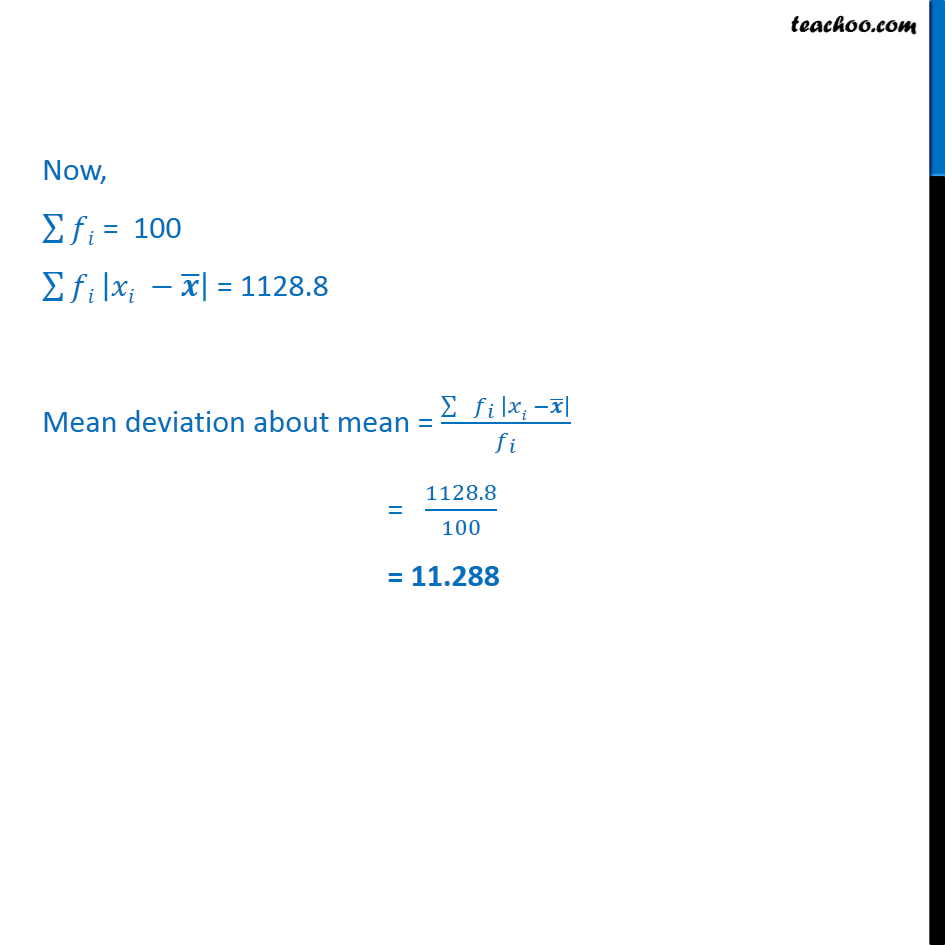Mean deviation about mean - Continuous frequency distibution

Chapter 13 Class 11 Statistics
Concept wiseLearn in your speed, with individual attention - Teachoo Maths 1-on-1 Class

### Transcript

Ex 13.1, 10 Find the mean deviation about the mean for the data Now, ∑128▒𝑓𝑖 = 100 ∑128▒𝑓𝑖 |𝑥𝑖 −𝒙 ̅ | = 1128.8 Mean deviation about mean = (∑▒〖 𝑓_𝑖 |𝑥𝑖 −𝒙 ̅ | 〗)/𝑓_𝑖 = 1128.8/100 = 11.288